Like   Tweet   Pin
 /* styles */ Hi everyone and best wishes for the new year 2018! My winter news update shows a busy start for 2018 with a variety of exhibitions. The news begins with a presentation which includes two versions of my steel panel portrait of Scottish poet Robert Burns: one which is a flat suspended steel panel and another which is convex and free-standing on a base. The newsletter ends with another convex freestanding/suspended steel panel portrait – though of a completely different subject!!!
 table div table+table+table div table{width:100%;padding:0}table div table+table+table div table img{width:96.23%;padding:0;float:none}table div table+table+table div table td{width:100%;padding:0 1.88% 18px}/* styles */## ‘BURNS UNBROKE‘ Edinburgh

 /* styles */ 25 January – 10 March Summerhall, Edinburgh, 11.00am – 6.00pm, free entry with ARTRUIST, Edinburgh - more info - press release ‘Burns Unbroke‘ is a pilot for a contemporary multi-arts festival, exploring new interpretations of the life and work of Robert Burns, supported by Creative Scotland. It features the work of over 30 visual artists, across eleven galleries, an Alternative Burns night, a Flyting competition, a series of Whisky Masterclasses, music and children’s workshops.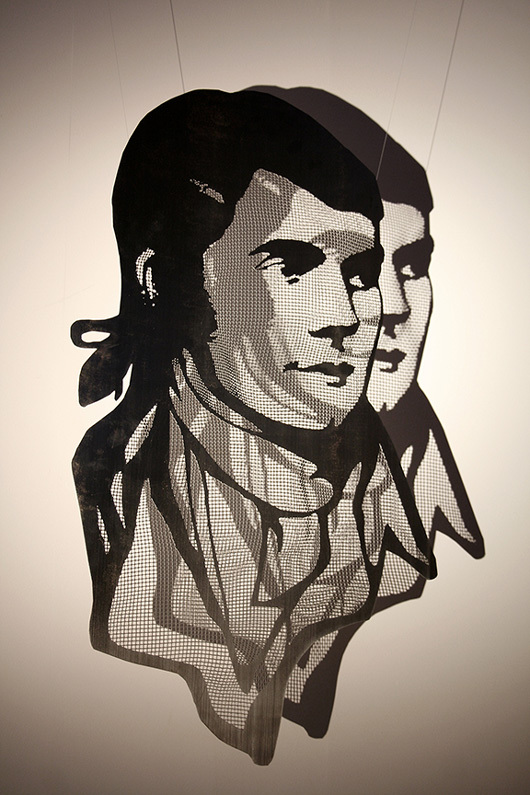‘BURNS‘ steel panel sculpture in limited edition, suspended, 104 cm height, freestanding 110 cm height.
 table div table+table+table+table+table+table+table div table,table.module-6{width:37.74%;float:right;padding:0}table div table+table+table+table+table+table+table div table a{border:0 none;text-decoration:none}table div table+table+table+table+table+table+table div table img{width:100%!important;border:0 none;text-decoration:none}table div table+table+table+table+table+table+table div table td{width:100%;padding:0 0 20px 20px}/* styles */ The title of ‘Burns Unbroke‘ was inspired by the epigraph which prefixed Robert Burns’s first publication in 1786: The simple Bard, unbroke by rules of art, He pours the wild effusions of the heart; And if inspir’d ’tis Nature’s pow’rs inspire; Her’s all the melting thrill, and her’s the kindling fire. Robert Burns Day 25th January 2018!
 table div table+table+table+table+table+table+table+table div table{width:100%;padding:0}table div table+table+table+table+table+table+table+table div table img{width:96.23%;padding:0;float:none}table div table+table+table+table+table+table+table+table div table td{width:100%;padding:0 1.88% 18px}/* styles */## ‘CONTEMPO‘ Munich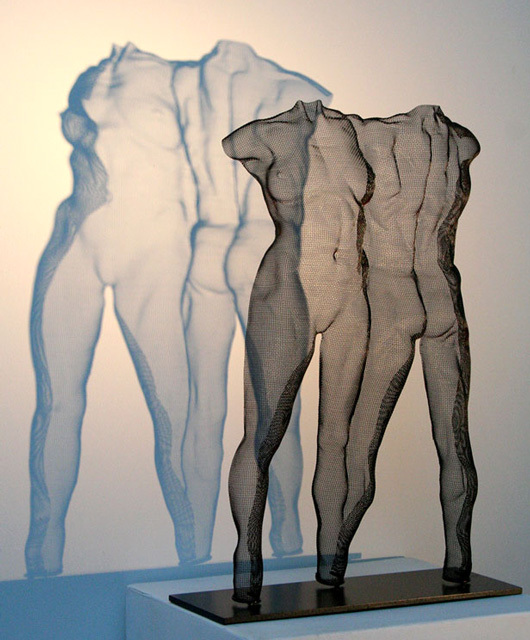‘IZUS‘ 2016, freestanding, 52cm height.
 table div table+table+table+table+table+table+table+table+table+table+table div table,table.module-10{width:50.94%;float:right;padding:0}table div table+table+table+table+table+table+table+table+table+table+table div table a{border:0 none;text-decoration:none}table div table+table+table+table+table+table+table+table+table+table+table div table img{width:100%!important;border:0 none;text-decoration:none}table div table+table+table+table+table+table+table+table+table+table+table div table td{width:100%;padding:0 0 20px 20px}/* styles */ 02 Februar – 07 Apr 2018 Galerie Hegemann Hackenstraße 5 Munich, Germany
 /* styles */ This exhibition presents a broad spectrum of high-quality international contemporary art works: ‘CONTEMPO‘ features unique sculptures from Scottish sculptor DAVID BEGBIE, Italian artist RABARAMA and Dutch steel sculptor RONALD A. WESTERHUIS which interact dynamically with the expressive paintings from English painter NIGEL COX, Swiss artist FRANK FISCHER and German painter IGOR OLEINIKOV. Contact me or Gallery Hegemann for the private view invitation 2nd February where I will attend.
 table div table+table+table+table+table+table+table+table+table+table+table+table+table div table{width:100%;padding:0}table div table+table+table+table+table+table+table+table+table+table+table+table+table div table img{width:96.23%;padding:0;float:none}table div table+table+table+table+table+table+table+table+table+table+table+table+table div table td{width:100%;padding:0 1.88% 18px}/* styles */## ‘First Art Fair‘ Amsterdam

 table div table+table+table+table+table+table+table+table+table+table+table+table+table+table+table div table td,table.module-14{width:100%;padding:0}table div table+table+table+table+table+table+table+table+table+table+table+table+table+table+table div table{width:100%;float:none;margin-left:auto;margin-right:auto;padding:0}table div table+table+table+table+table+table+table+table+table+table+table+table+table+table+table div table a{border:0 none;text-decoration:none}table div table+table+table+table+table+table+table+table+table+table+table+table+table+table+table div table img{width:100%!important;border:0 none;text-decoration:none}/* styles */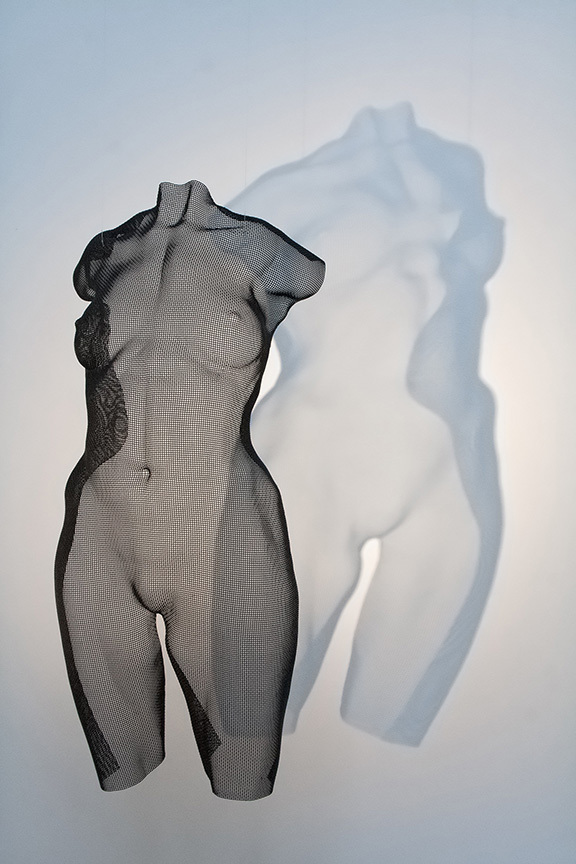‘SENSU‘, suspended, 64 cm height
 /* styles */ 17 - 21 January 2018 Passenger Terminal Amsterdam, (Piet Heinkade 27, 1019 BR Amsterdam) with Van Loon Galleries, Stand 18, The Netherlands ‘SENSU‘ and other examples of contemporary body sculptures will be exhibited. More info: FAF and www.vanloongalleries.com
 table div table+table+table+table+table+table+table+table+table+table+table+table+table+table+table+table+table+table div table{width:100%;padding:0}table div table+table+table+table+table+table+table+table+table+table+table+table+table+table+table+table+table+table div table img{width:96.23%;padding:0;float:none}table div table+table+table+table+table+table+table+table+table+table+table+table+table+table+table+table+table+table div table td{width:100%;padding:0 1.88% 18px}/* styles */## ‘ARTE FIERA‘ Bergamo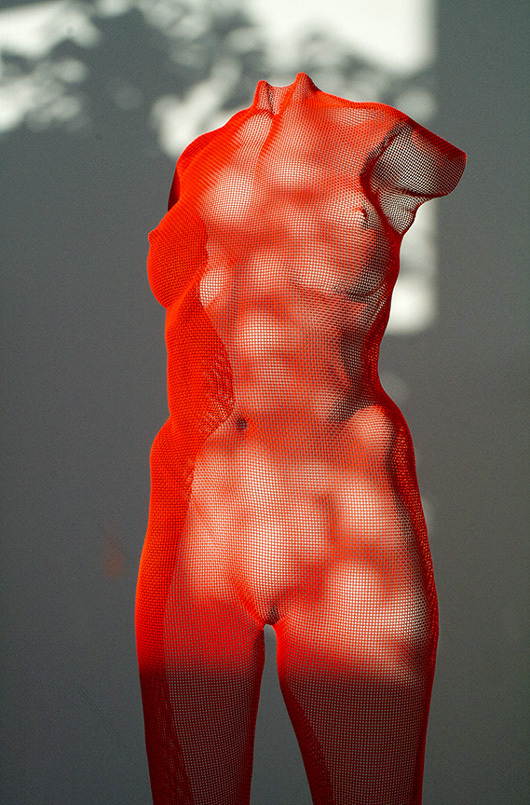‘FEME‘ freestanding, 92.5 cm height
 table div table+table+table+table+table+table+table+table+table+table+table+table+table+table+table+table+table+table+table+table+table div table,table.module-20{width:47.17%;float:right;padding:0}table div table+table+table+table+table+table+table+table+table+table+table+table+table+table+table+table+table+table+table+table+table div table a{border:0 none;text-decoration:none}table div table+table+table+table+table+table+table+table+table+table+table+table+table+table+table+table+table+table+table+table+table div table img{width:100%!important;border:0 none;text-decoration:none}table div table+table+table+table+table+table+table+table+table+table+table+table+table+table+table+table+table+table+table+table+table div table td{width:100%;padding:0 0 20px 20px}/* styles */ 13 Jan - 15 Jan BAF, Bergamo Vecchiato Arte will be exhibiting a selection of my sculpture. Pictured: FEME in red colour finish.
 table div table+table+table+table+table+table+table+table+table+table+table+table+table+table+table+table+table+table+table+table+table+table div table{width:100%;padding:0}table div table+table+table+table+table+table+table+table+table+table+table+table+table+table+table+table+table+table+table+table+table+table div table img{width:96.23%;padding:0;float:none}table div table+table+table+table+table+table+table+table+table+table+table+table+table+table+table+table+table+table+table+table+table+table div table td{width:100%;padding:0 1.88% 18px}/* styles */## ‘IN THE HEAT OF THE NIGHT‘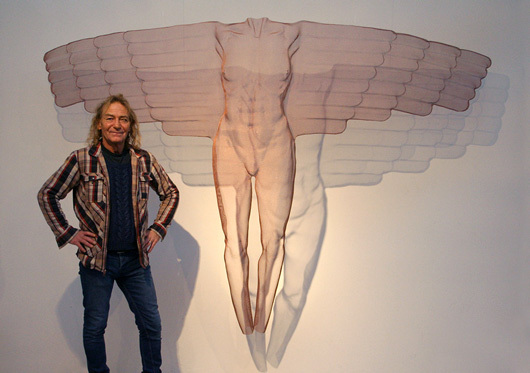‘IKARUS‘ 2017, suspended, 180 cm height
 /* styles */ 03 Dec 2017 - 04 Feb 2018 Königswinter near Bonn, Germany 20 Artists - 60 Artworks / 20 Künster - 60 Werke Continuum Gallery - expressive glass & contemporary art, presents a selection of my work including this large bronzemesh angel titled ‘IKARUS‘.
 table div table+table+table+table+table+table+table+table+table+table+table+table+table+table+table+table+table+table+table+table+table+table+table+table+table+table div table td,table.module-25{width:100%;padding:0}table div table+table+table+table+table+table+table+table+table+table+table+table+table+table+table+table+table+table+table+table+table+table+table+table+table+table div table{width:100%;float:none;margin-left:auto;margin-right:auto;padding:0}table div table+table+table+table+table+table+table+table+table+table+table+table+table+table+table+table+table+table+table+table+table+table+table+table+table+table div table a{border:0 none;text-decoration:none}table div table+table+table+table+table+table+table+table+table+table+table+table+table+table+table+table+table+table+table+table+table+table+table+table+table+table div table img{width:100%!important;border:0 none;text-decoration:none}/* styles */
 table div table+table+table+table+table+table+table+table+table+table+table+table+table+table+table+table+table+table+table+table+table+table+table+table+table+table+table div table{width:100%;padding:0}table div table+table+table+table+table+table+table+table+table+table+table+table+table+table+table+table+table+table+table+table+table+table+table+table+table+table+table div table img{width:96.23%;padding:0;float:none}table div table+table+table+table+table+table+table+table+table+table+table+table+table+table+table+table+table+table+table+table+table+table+table+table+table+table+table div table td{width:100%;padding:0 1.88% 18px}/* styles */## Latest Creation

 /* styles */ Finally my latest creation ‘SPACEMAN‘ 2017 is a commissioned portrait, a painted convex steel panel sculpture of the first man on the moon! (See all images).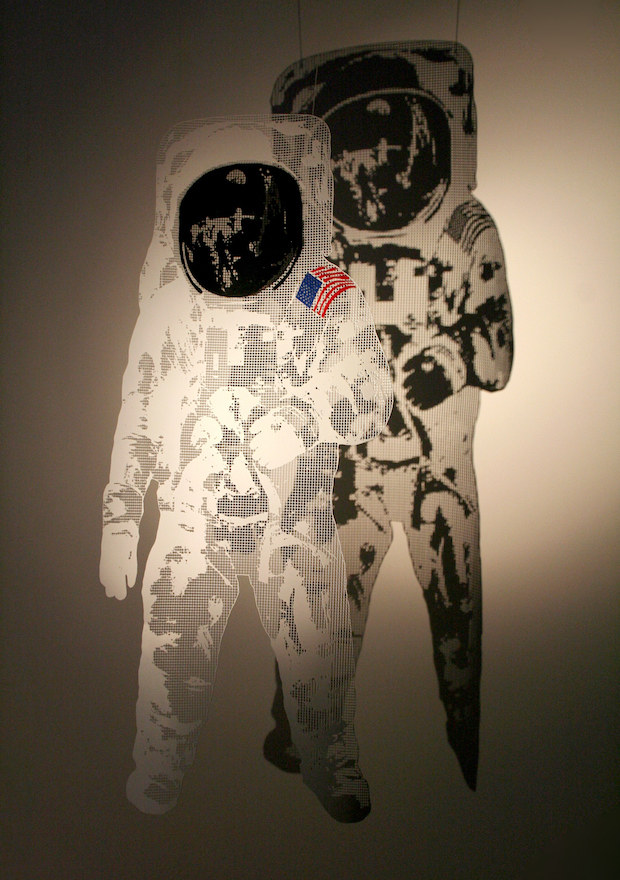‘SPACEMAN‘ 2017, freestanding or suspended, 120 cm height.
 table div table+table+table+table+table+table+table+table+table+table+table+table+table+table+table+table+table+table+table+table+table+table+table+table+table+table+table+table+table+table+table div table{width:100%;padding:0}table div table+table+table+table+table+table+table+table+table+table+table+table+table+table+table+table+table+table+table+table+table+table+table+table+table+table+table+table+table+table+table div table img{width:96.23%;padding:0;float:none}table div table+table+table+table+table+table+table+table+table+table+table+table+table+table+table+table+table+table+table+table+table+table+table+table+table+table+table+table+table+table+table div table td{width:100%;padding:0 1.88% 18px}/* styles */table div table+table+table+table+table+table+table+table+table+table+table+table+table+table+table+table+table+table+table+table+table+table+table+table+table+table+table+table+table+table+table+table+table div table{width:100%;padding:0}table div table+table+table+table+table+table+table+table+table+table+table+table+table+table+table+table+table+table+table+table+table+table+table+table+table+table+table+table+table+table+table+table+table div table img{width:96.23%;padding:0;float:none}table div table+table+table+table+table+table+table+table+table+table+table+table+table+table+table+table+table+table+table+table+table+table+table+table+table+table+table+table+table+table+table+table+table div table td{width:100%;padding:0 1.88% 18px}/* styles *//* styles */ Why do I receive this? We are writing to you as we understand you'd like to receive David Begbie news from time to time. We hope no-one is receiving this mail-out who does not wish to. If so, accept our many apologies ... and please reply by ‘unsubscribe’ below - thank you.
 table div table+table+table+table+table+table+table+table+table+table+table+table+table+table+table+table+table+table+table+table+table+table+table+table+table+table+table+table+table+table+table+table+table+table+table div table{width:100%;padding:0}table div table+table+table+table+table+table+table+table+table+table+table+table+table+table+table+table+table+table+table+table+table+table+table+table+table+table+table+table+table+table+table+table+table+table+table div table img{width:96.23%;padding:0;float:none}table div table+table+table+table+table+table+table+table+table+table+table+table+table+table+table+table+table+table+table+table+table+table+table+table+table+table+table+table+table+table+table+table+table+table+table div table td{width:100%;padding:0 1.88% 18px}/* styles */table div table+table+table+table+table+table+table+table+table+table+table+table+table+table+table+table+table+table+table+table+table+table+table+table+table+table+table+table+table+table+table+table+table+table+table+table div table,table.module-35{width:64.53%;float:right;padding:0}table div table+table+table+table+table+table+table+table+table+table+table+table+table+table+table+table+table+table+table+table+table+table+table+table+table+table+table+table+table+table+table+table+table+table+table+table div table a{border:0 none;text-decoration:none}table div table+table+table+table+table+table+table+table+table+table+table+table+table+table+table+table+table+table+table+table+table+table+table+table+table+table+table+table+table+table+table+table+table+table+table+table div table img{width:100%!important;border:0 none;text-decoration:none}table div table+table+table+table+table+table+table+table+table+table+table+table+table+table+table+table+table+table+table+table+table+table+table+table+table+table+table+table+table+table+table+table+table+table+table+table div table td{width:100%;padding:0 0 20px 20px}/* styles */ Wiremesh kindly provided by United Wire, Edinburgh